##### Hot Keywords

TopIntell Robot 2022;2(1):89-104. 10.20517/ir.2022.02 © The Author(s) 2022.
Open Access Research Article

# An open-closed-loop iterative learning control for trajectory tracking of a high-speed 4-dof parallel robot

School of Mechanical Engineering, Dalian University of Technology, Dalian 116024, Liaoning, China

Correspondence to: Assoc. Prof./Dr. Guanglei Wu, School of Mechanical Engineering, Dalian University of Technology, No.2 Linggong Road, Ganjingzi District, Dalian 116024, Liaoning, China. E-mail: gwu@dlut.edu.cn

Views:767 | Downloads:168 | Cited:0 | Comments:0 | :3
Academic Editors: Simon X. Yang, Tao Ren | Copy Editor: Jia-Xin Zhang | Production Editor: Jia-Xin Zhang

© The Author(s) 2022. Open Access This article is licensed under a Creative Commons Attribution 4.0 International License (https://creativecommons.org/licenses/by/4.0/), which permits unrestricted use, sharing, adaptation, distribution and reproduction in any medium or format, for any purpose, even commercially, as long as you give appropriate credit to the original author(s) and the source, provide a link to the Creative Commons license, and indicate if changes were made.

## Abstract

Precise control is of importance for robots, whereas, due to the presence of modeling errors and uncertainties under the complex working environment, it is difficult to obtain an accurate dynamic model of the robot, leading to decreased control performances. This work presents an open-closed-loop iterative learning control applied to a four-limb parallel Schönflies-motion robot, aiming to improve the tracking accuracy with high movement, in which the controller can learn from the iterative errors to make the robot end-effector approximate to the expected trajectory. The control algorithm is compared with classical D-ILC, which is illustrated along with an industrial trajectory of pick-and-place operation. External repetitive and non-repetitive disturbances are added to verify the robustness of the proposed approach. To verify the overall performance of the proposed control law, multiple trajectories within the workspace, different working frequencies for a prescribed trajectory, and different design methods are selected, which show the effectiveness and the generalization ability of the designed controller.

## 1. INTRODUCTION

With the rapid development of robotic technology, robots have found their industrial applications in many fields to replace a large amount of manpower. Among their applications, material handling is an important aspect, in which the Delta and SCARA robots are extensively deployed. Compared to serial robots, parallel robots have received more attention thanks to their high speed, high stiffness-to-weight ratio, and low inertia, dedicated to pick-and-place operations (PPOs) with high dynamic movements. For instance, Figure 1 depicts a four-degree-of-freedom (4-dof) robot of this family suitable for PPO. Accordingly, the design of a control system for the robot under study is the focus of this work, since precise control is of importance, in particular for such a robot working with highly frequent switching of joint motions.

In the control design, classical model-free controller techniques, such as PID and PD controls, have been extensively adopted by industrial robots due to their simplicity and ease of implementation. However, these controllers are not applicable to parallel robots due to the highly nonlinear coupled characteristics. In this light, some control methods, such as torque feedforward control, computed torque control, sliding mode control[5, 6], etc., have been proposed to improve the control quality for parallel robots. Although those methods overcome some problems, such as trajectory tracking accuracy, other problems (i.e., increased computational burden and requirement of an accurate dynamic model) arise. Taking the characteristics of repetitive tasks for most parallel robots into consideration, it turns out that iterative learning control (ILC) is suitable for controlling the parallel robots, as ILC can benefit robot control from the system repeatability, wherein ILC makes use of the last output motion of the robot end-effector to obtain control inputs that can track the desired trajectory repeatedly.

ILC was first proposed in 1978, but it did not attract the attention of researchers until 1984 because of language restrictions. Over several decades, ILC has been developed and improved with numerous variants. One example is the ILC with a P-type switching surface using a proportional structure, which can effectively cope with external disturbances. Compared with the sliding mode surface, this controller is able to remove the chattering in the control process. It has been used for mobile robots to improve the robustness of path tracking against the presence of initial shifts, but it introduced a large trajectory tracking error and had a poor convergence effect. The D-type ILC is proposed with an initial condition algorithm to specify the initial state value in each iteration automatically. However, a lot of jittering occurs in the control torque, leading to damage to the actuator and some other robotic components. Sequentially, a modified D-type ILC was designed to effectively avoid the jitter and glitch for enhanced convergence accuracy, compared to the conventional D-type one. By means of the filter, another D-type ILC method with a unit-gain derivative is proposed to compensate for the unexpected high gain of the conventional derivative at high frequency, wherein the desired phase compensation can be realized within a designated frequency band.

Despite the advantages of the above-mentioned ILC methods, neither P- nor D-type learning laws can make full use of system information. In the control law, P- and D-type gains not only play a role in learning gain but also take the task of accomplishment of the feedback in the control system[13, 14]. However, it is difficult to achieve the compatibility between feedback stability and learning convergence. Alternatively, PD-type ILCs are deployed in parallel robots. For instance, an open-loop PD-type ILC algorithm was proposed for a class of nonlinear time-varying systems with control delay and arbitrary initial value. In this manner, the learning convergence curve is not smooth, although it solves the problem of initial shift. The robustness of the controller can be ensured by designing a robust term, aiming at the control of a 3-dof permanent magnet spherical actuator. Open-loop PD-type ILCs have also been applied in the Delta robot; however, the test on the controller showed that convergence requires a number of iterations and plenty of computation time, i.e., an unacceptable computational burden in real applications. To speed up the convergence of the controller, the constant gain of the PD control can be changed to a time-varying one, but this introduces glitches during the convergence procedure. Alternatively, an adaptive controller can be integrated, where the controller gain is defined as a function of the number of iterations; sequentially, both the position and velocity tracking errors can be monotonically and rapidly reduced. In addition, to realize the automatic tuning of a controller, a method with generalization capabilities is proposed in that can effectively tune the parameters to improve the trajectory tracking accuracy for robots. Besides, ILC can also be applied in repetitive rehabilitation training, in which a high-order ILC can improve the transient performance and decrease the steady-state error, compared to traditional PID controllers. Since ILC is equivalent to an integrator along with the iterations, it is sensitive to external disturbances. The focus of this work is the design of an ILC considering disturbances for high-speed parallel robots for a pick-and-place application.

In the practical application of industrial robots, classical PD control is still the mainstream algorithm, and studies on the iterative learning theory applied to control of parallel robots have not been extensively reported. Consequently, the present work is to illustrate the effectiveness and feasibility of such algorithms for parallel robots. In this paper, an open-closed-loop PD-type ILC method is proposed and illustrated with a parallel robot producing Schönflies motion. The proposed ILC law consists of classical PD control and ILC. The iterative learning term can be regarded as feedforward compensation, which can use the information stored in the last movement. The PD control part belongs to the feedback item and performs real-time compensation. The controller convergence is proved based on Q operator theory, and the tracking performance is tested by tracking a pick-and-place trajectory and compared with the classical D-ILC controller. Moreover, different trajectories and working frequencies are selected to verify the effectiveness of the controller.

## 2. ROBOT STRUCTURE AND DYNAMIC MODEL

Figure 2 depicts the detailed CAD model of the robot shown in Figure 1, which is composed of a mounting frame, a screw-pair-based moving platform, and four identical limbs. Each limb consists of a big (inner) arm and a small (outer) arm. A drive motor and a reducer are installed on the rotating shaft of the big arm. The outer arm is composed of two carbon fiber rods in a $$\pi$$ -shape. The inner and outer arms are connected by ball joints, as well as the connection between the outer arm and the mobile platform. The mobile platform can be split into two subparts, i.e., upper and lower sub-platforms. Through the helix joint, the rotation in the vertical direction of the end-effector can be generated by the differential motion of the two sub-platforms.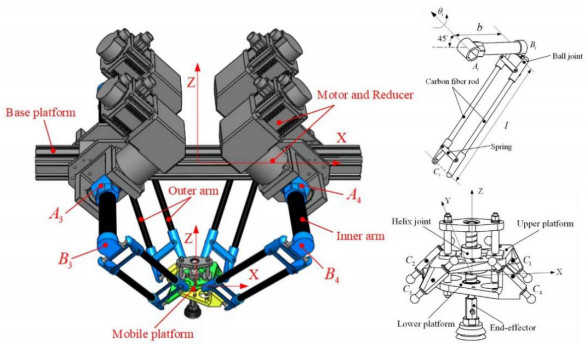Figure 2. CAD model of the 4-dof robot with a revolute-spherical-spherical limb and a screw pair-based mobile platform.

The kinematics and dynamics of the robot have been well documented in the previous work, which is revisited by skipping the details. When ignoring un-modeled errors and external disturbance, the dynamic model of the robot can be expressed as:

\begin{align} \vec{\mathit{\boldsymbol{\tau}}}=\mathit{\boldsymbol{M}} (\vec{\mathit{\boldsymbol{q}}})\vec{\ddot{\mathit{\boldsymbol{q}}}}+\mathit{\boldsymbol{C}}( \vec{{\mathit{\boldsymbol{q}}}}, \vec{\dot{{\mathit{\boldsymbol{q}}}}} ) \vec{\dot{{\mathit{\boldsymbol{q}}}}}+\vec{\mathit{\boldsymbol{G}}} (\vec{{\mathit{\boldsymbol{q}}}}) \end{align}

with

\begin{align} {\mathit{\boldsymbol{M}}}({\vec{{\mathit{\boldsymbol{q}}}}}) & = {\mathit{\boldsymbol{J}}}_{\rm{low}}^{\rm T} {\mathit{\boldsymbol{M}}}_{\rm{p, low}} {\mathit{\boldsymbol{J}}}_{\rm{low}} +{\mathit{\boldsymbol{J}}}_{\rm{up}}^{\rm T} {\mathit{\boldsymbol{M}}}_{\rm{p, up}} {\mathit{\boldsymbol{J}}}_{\rm{up}} +{\mathit{\boldsymbol{I}}}_{\rm b} \end{align}

\begin{align} {\mathit{\boldsymbol{C}}}({\vec{{\mathit{\boldsymbol{q}}}}, \vec{\dot{{\mathit{\boldsymbol{q}}}}}}) & ={\mathit{\boldsymbol{J}}}_{\rm{low}}^{\rm T} {\mathit{\boldsymbol{M}}}_{\rm{p, low}} \dot{J}_{\rm{low}} +{\mathit{\boldsymbol{J}}}_{\rm{up}}^{\rm T} {\mathit{\boldsymbol{M}}}_{\rm{p, up}} \dot{{\mathit{\boldsymbol{J}}}}_{\rm{up}} \end{align}

\begin{align} \vec{{\mathit{\boldsymbol{G}}}}({\vec{{\mathit{\boldsymbol{q}}}}}) & =-{\mathit{\boldsymbol{J}}}_{\rm{low}}^{\rm T} {\mathit{\boldsymbol{M}}}_{\rm{p, low}} \vec{{\mathit{\boldsymbol{g}}}}-{\mathit{\boldsymbol{J}}}_{\rm{up}}^{\rm T} {\mathit{\boldsymbol{M}}}_{\rm{p, up}} \vec{{\mathit{\boldsymbol{g}}}}-{\mathit{\boldsymbol{M}}}_{\rm b} \vec{{\mathit{\boldsymbol{g}}}} \end{align}

where $$\vec{\mathit{\boldsymbol{\tau}}}\in R^{4}$$ is the driving torque and $$\vec{\dot{\mathit{\boldsymbol{q}}}}, \vec{\ddot{\mathit{\boldsymbol{q}}}}\in R^{4}$$ represent the joint angular velocity and acceleration, respectively. Moreover, $$\mathit{\boldsymbol{M}}(\vec{\mathit{\boldsymbol{q}}})\in R^{4\times 4}$$ is the inertia matrix, $${\mathit{\boldsymbol{C}}}( \vec{{\mathit{\boldsymbol{q}}}}, \vec{\dot{{\mathit{\boldsymbol{q}}}}} )\in R^{4\times 4}$$ is a vector resulting from Coriolis and centrifugal forces, $$\vec{\mathit{\boldsymbol{G}}}({\vec{\mathit{\boldsymbol{q}}}})\in R^{4}$$ represents gravity, and $$\mathit{\boldsymbol{I}}_{\rm b}$$ is the moment of inertia of inner arms. Jacobians $$\mathit{\boldsymbol{J}}_{\rm{up}}$$ and $$\mathit{\boldsymbol{J}}_{\rm{down}}$$ relate the motion of the upper and lower sub-platforms to the actuated joints, while $$\dot{\mathit{\boldsymbol{J}}}_{\rm{up}}$$ and $$\dot{\mathit{\boldsymbol{J}}}_{\rm{down}}$$ , respectively, represent their derivatives with respect to time. In addition, $$\mathit{\boldsymbol{M}}_{\rm b}$$ , $$\mathit{\boldsymbol{M}}_{\rm{p, up}}$$ , and $$\mathit{\boldsymbol{M}}_{\rm{p, down}}$$ are the mass matrices of the inner arm and the upper and lower sub-platforms. The detailed modeling procedure can be found in Ref. The main geometric and dynamic parameters of the parallel robot are listed in Table 1.

Table 1

Geometric and dynamic parameters of the robot

 Parameters Value Length of inner arm 0.296 m Length of outer arm 0.600 m Mass of upper platform 0.855 kg Mass of lower platform 1.080 kg Mass of inner arm 0.842 kg Mass of outer arm 0.073 kg

## 3. ITERATIVE LEARNING CONTROLLER DESIGN

Prior to the ILC design for the robot, the following properties generalized to the robotic manipulators are considered.

Property 1. The inertia matrix is bounded and positive definite, thus $$\exists \delta >0, \zeta >0$$ satisfies the following inequalities:

\begin{align} 0<\delta <\| \mathit{\boldsymbol{M}} (\vec{\mathit{\boldsymbol{q}}}_{k}, t) \|<\zeta \end{align}

Property 2. The inertia matrix satisfies the global Lipschitz condition; therefore, a positive constant $$L$$ exists that meets:

\begin{align} \| {\mathit{\boldsymbol{M}}(\vec{\mathit{\boldsymbol{q}}}_{k} , t)-\mathit{\boldsymbol{M}}(\vec{\mathit{\boldsymbol{q}}}_{k-1}, t)} \|\leq L\| {\vec{\mathit{\boldsymbol{q}}}_{k} (t)-\vec{{\mathit{\boldsymbol{q}}}}_{k-1} (t)} \| \end{align}

where $$k$$ represents the number of iterations and $$\vec{\mathit{\boldsymbol{q}}}$$ is the angular displacement of the joint.

Property 3. Coriolis, centrifugal, and gravitational force matrices meet the equation $${\mathit{\boldsymbol{C}}}(\vec{{\mathit{\boldsymbol{q}}}}_{k}, \vec{\dot{{\mathit{\boldsymbol{q}}}}}_{k})\vec{\dot{{\mathit{\boldsymbol{q}}}}}_{d} +\vec{{\mathit{\boldsymbol{G}}}}(\vec{{\mathit{\boldsymbol{q}}}}_{k})=\bm \varphi (\vec{{\mathit{\boldsymbol{q}}}}_{k}, \vec{\dot{{\mathit{\boldsymbol{q}}}}}_{k})\vec{\bm\gamma}_{k} (t)$$ , where $$\mathit{\boldsymbol{\varphi}} (\vec{\mathit{\boldsymbol{q}}}_{k} , \vec{\dot{\mathit{\boldsymbol{q}}}}_{k})\in R^{n\times m}$$ is a regression matrix and $$\vec{\mathit{\boldsymbol{\gamma}}}_{k} (t)\in R^{m\times 1}$$ is a vector of unknown parameters regarding the robot.

Moreover, the following reasonable assumptions are made.

Assumption 1. The system can meet the alignment condition, i.e.,$$\vec{\mathit{\boldsymbol{q}}}_{k} (0)=\vec{\mathit{\boldsymbol{q}}}_{d} (0)$$ , $$\vec{\dot{\mathit{\boldsymbol{q}}}}_{k} (0)=\vec{\dot{\mathit{\boldsymbol{q}}}}_{d} (0)$$ . The desired joint position trajectory, namely, $$\vec{\mathit{\boldsymbol{q}}}_{d}$$ , and its $$n$$ th derivatives are bounded, namely, $$\forall t\in [0, T]$$ , $$\forall k\in Z_+$$ .

Assumption 2. The external disturbance of the robot is bounded and is subject to a positive constant:

\begin{align} \sup \| \vec{\mathit{\boldsymbol{d}}}_{k} (t) \|\leq l \end{align}

In view of the nonlinear time-varying robotic system with repetitive work over a finite interval time $$t\in [0, T]$$ , an open-closed loop PD-ILC law is designed. This algorithm belongs to the feedback–feedforward control law, which can make full use of the effective information stored in the system for learning and can ensure that the output variables converge to the bounded threshold of desired values.

The specific expression is written as follows:

\begin{align} \vec{\bm \tau}_{k+1} (t)=\vec{\bm \tau}_{k} (t)+\vec{\mathit{\boldsymbol{\tau}}}_{\rm{fore}} +\vec{\bm \tau}_\rm{back} \end{align}

where $$\vec{\mathit{\boldsymbol{\tau}}}$$ is the driving torque and $$k$$ is the number of iterations. Moreover, $$\vec{\mathit{\boldsymbol{\tau}}}_{\rm{fore}}$$ is the feedforward control input, written as:

\begin{align} \vec{\mathit{\boldsymbol{\tau}}}_{\rm{fore}} ={\mathit{\boldsymbol{L}}}_{p} \vec{{\mathit{\boldsymbol{e}}}}_{k} (t)+{\mathit{\boldsymbol{L}}}_{d} \vec{\dot{{\mathit{\boldsymbol{e}}}}}_{k} (t) \end{align}

where $$\mathit{\boldsymbol{L}}_{p}, \mathit{\boldsymbol{L}}_{d}$$ are symmetric positive definite gain matrices for the feedforward control and $$\vec{{\mathit{\boldsymbol{e}}}}_{k} =\vec{{\mathit{\boldsymbol{q}}}}_{k} -\vec{{\mathit{\boldsymbol{q}}}}_{d}$$ and $$\vec{\dot{{\mathit{\boldsymbol{e}}}}}_{k} =\vec{\dot{{\mathit{\boldsymbol{q}}}}}_{k} -\vec{\dot{{\mathit{\boldsymbol{q}}}}}_{d}$$ represent the joint errors in terms of angular displacement and angular velocity, respectively, in the $$k$$ th iteration.

The feedback control $$\vec{\bm \tau}_\rm{back}$$ takes the following form:

\begin{align} \vec{\mathit{\boldsymbol{\tau}}}_\rm{back} =(1-\alpha) {\mathit{\boldsymbol{L}}}_{p} \vec{{\mathit{\boldsymbol{e}}}}_{k+\mathit{\boldsymbol{1}}} (t)+(1-\beta) {\mathit{\boldsymbol{L}}}_{d} \vec{\dot{{\mathit{\boldsymbol{e}}}}}_{k+\mathit{\boldsymbol{1}}} (t) \end{align}

where $$\alpha$$ and $$\beta$$ are gain coefficients of the controller.

The scheme of the proposed controller is displayed in Figure 3. It can be seen that the information obtained in the $$k$$ th iteration can be regarded as the feedforward part. The current joint errors, namely, the information obtained in the ($$k+1$$ )th iteration, constitute the feedback part of the control law. Under the condition that the control target and external environment remain unchanged, the target task is repeatedly executed, and the response of the system is identical to the feedforward information. When the system deviates from the desired trajectory, the feedback term will compensate the motion errors.

## 4. CONVERGENCE ANALYSIS OF THE CONTROLLER

To prove the convergence of proposed controller, the following two lemmas are introduced as the fundamentals.

Lemma 1. With $$\forall \vec{\mathit{\boldsymbol{x}}}, \vec{\mathit{\boldsymbol{y}}}\in C_{r} [0, T], t\in [0, T]$$ , assuming that the operator $$\vec{\mathit{\boldsymbol{Q}}}:C_{r} [0, T]\to C_{r} [0, T]$$ meets global Lipschitz condition, one obtains the following two outcomes.

(1) For $$\forall \vec{\mathit{\boldsymbol{y}}}\in C_{r} [0, T]$$ , there is a unique $$\vec{\mathit{\boldsymbol{x}}}\in C_{r} [0, T]$$ that holds:

\begin{align} \vec{\mathit{\boldsymbol{x}}} (t)+\vec{\mathit{\boldsymbol{Q}}}(\vec{\mathit{\boldsymbol{x}}})(t)=\vec{\mathit{\boldsymbol{y}}} (t) , \; \forall t\in [0, T] \end{align}

(2) Defining the operator $$\vec{\bar{\mathit{\boldsymbol{Q}}}}$$ yields

\begin{align} \vec{\bar{\mathit{\boldsymbol{Q}}}}(\vec{\mathit{\boldsymbol{y}}})(t)=\vec{\mathit{\boldsymbol{Q}}}(\vec{\mathit{\boldsymbol{x}}})(t) , \; \forall \vec{\mathit{\boldsymbol{y}}}\in C_{r} [0, T] \end{align}

where $$\vec{\mathit{\boldsymbol{x}}}\in C_{r} [0, T]$$ is the only solution to the first outcome, and there exists a constant $$M_{1} >0$$ subject to:

\begin{align} \| \vec{\bar{\mathit{\boldsymbol{Q}}}}(\vec{\mathit{\boldsymbol{x}}})(t) \|\leq M_{1} \left(q+\int_0^t \| \vec{\mathit{\boldsymbol{y}}}(s) \| {{{\rm{d}}}} s\right) \end{align}

Lemma 2. Assuming that the sequence $$\{{b_{k}} \}_{k\geq 0}$$ , $$b_{k} \geq 0$$ , converges to zero, the operator $$\vec{\mathit{\boldsymbol{Q}}}_{k} :C_{r} [0, T]\to C_{r} [0, T]$$ will meet

\begin{align} \| {\vec{\mathit{\boldsymbol{Q}}}_{k} (\vec{\mathit{\boldsymbol{x}}})(t)} \|\leq M \left(b_{k} +\int_0^t {\| {\vec{\mathit{\boldsymbol{x}}}(s)} \|} {{{\rm{d}}}} s+\sigma\right) \end{align}

where $$\sigma >0$$ and $$M\geq 1$$ are constants. Assuming that $$\mathit{\boldsymbol{P}} (t)$$ is a $$r\times r$$ continuous function matrix, the operator $$\vec{\mathit{\boldsymbol{P}}}:C_{r} [0, T]\to C_{r} [0, T]$$ satisfies $$\vec{\mathit{\boldsymbol{P}}}(\vec{\mathit{\boldsymbol{x}}})(t)=\mathit{\boldsymbol{P}}(t)\vec{\mathit{\boldsymbol{x}}}(t)$$ . If $$\rho <1$$ , $$\rho$$ being the spectral radius of $$\vec{\mathit{\boldsymbol{P}}}$$ , for $$\forall t\in [0, T]$$ , there exists

\begin{align*} \lim _{n\to \infty} (\vec{\mathit{\boldsymbol{P}}}+\vec{\mathit{\boldsymbol{Q}}}_{\rm n})(\vec{\mathit{\boldsymbol{P}}}+\vec{\mathit{\boldsymbol{Q}}}_{n-1})\cdots (\vec{\mathit{\boldsymbol{P}}}+\vec{\mathit{\boldsymbol{Q}}}_{0})(\vec{\mathit{\boldsymbol{x}}})(t)=0 \end{align*}

For the parallel robot under study, the state variables $$\vec{\mathit{\boldsymbol{X}}}=[\vec{\mathit{\boldsymbol{x}}}_{1}, \vec{\mathit{\boldsymbol{x}}}_{2} ]_{8\times 1}^{\rm T}$$ are defined below:

\begin{align} \begin{cases} \vec{{\mathit{\boldsymbol{x}}}}_{1} =\vec{{\mathit{\boldsymbol{q}}}} \\ \vec{{\mathit{\boldsymbol{x}}}}_{2} =\vec{\dot{{\mathit{\boldsymbol{q}}}}} \\ \end{cases} \end{align}

Accordingly, the variable $$\vec{\bm\phi} (t, \vec{{\mathit{\boldsymbol{X}}}})_{4\times 1} =-{\mathit{\boldsymbol{M}}}^{-1}(\vec{{\mathit{\boldsymbol{q}}}})({{\mathit{\boldsymbol{C}}}({\vec{{\mathit{\boldsymbol{q}}}}, \vec{\dot{{\mathit{\boldsymbol{q}}}}}})\vec{\dot{{\mathit{\boldsymbol{q}}}}}+\vec{{\mathit{\boldsymbol{G}}}}({\vec{{\mathit{\boldsymbol{q}}}}})})$$ can be defined; thus, the dynamic model of the system can be expressed as:

\begin{align} \vec{\dot{{\mathit{\boldsymbol{X}}}}}= \begin{bmatrix} {\vec{\dot{{\mathit{\boldsymbol{x}}}}}_{1}} \\ {\vec{\dot{{\mathit{\boldsymbol{x}}}}}_{2}} \\ \end{bmatrix}= \begin{bmatrix} {\vec{\dot{{\mathit{\boldsymbol{q}}}}}} \\ {\vec{\bm\phi} (t, \vec{{\mathit{\boldsymbol{X}}}})} \\ \end{bmatrix}+ \begin{bmatrix} 0 \\ {\mathit{\boldsymbol{M}}}^{-1} (\vec{{\mathit{\boldsymbol{q}}}})\vec{\bm \tau} (t) \\ \end{bmatrix} \end{align}

As a consequence, the state equation of the robot can be obtained:

\begin{align} \begin{cases} \vec{\dot{{\mathit{\boldsymbol{X}}}}}=\vec{\mathit{\boldsymbol{\Phi}}} (t, \vec{{\mathit{\boldsymbol{X}}}})+{\mathit{\boldsymbol{B}}} (\vec{{\mathit{\boldsymbol{q}}}}, \vec{\dot{{\mathit{\boldsymbol{q}}}}})\vec{\bm \tau} (t) \\ \vec{{\mathit{\boldsymbol{Y}}}}={\mathit{\boldsymbol{C}}}\vec{{\mathit{\boldsymbol{X}}}} \end{cases} \end{align}

where $$\vec{\mathit{\boldsymbol{\Phi}}}(t, \vec{{\mathit{\boldsymbol{X}}}})_{8\times 1} =[\vec{\dot{{\mathit{\boldsymbol{q}}}}} \vec{\bm\phi} (t, \vec{{\mathit{\boldsymbol{X}}}})]_{8\times 1}^{\rm T}$$ , $${\mathit{\boldsymbol{B}}}(\vec{{\mathit{\boldsymbol{q}}}}, \vec{\dot{{\mathit{\boldsymbol{q}}}}})_{8\times 4} =[0, {\mathit{\boldsymbol{M}}}^{-1}(\vec{\mathit{\boldsymbol{q}}})]_{8\times 4}^{\rm T}$$ , and $${\mathit{\boldsymbol{C}}}=[0, {\mathit{\boldsymbol{I}}}]_{8\times 4}^{\rm T}$$ .

For the nonlinear system of Equation (17), based on the ILC law in Equation (8), if the system can meet the following condition,

\begin{align} \bm \rho ([{\mathit{\boldsymbol{I}}}+{\mathit{\boldsymbol{L}}}_{d} {\mathit{\boldsymbol{C}}}(t) {\mathit{\boldsymbol{B}}}(t)]^{-1}[{\mathit{\boldsymbol{I}}}-{\mathit{\boldsymbol{L}}}_{d} {\mathit{\boldsymbol{C}}}(t) {\mathit{\boldsymbol{B}}}(t)])<1, t\in [0, T] \end{align}

the trajectory tracking error of the dynamic system converges to a certain small range with the increasing iterations.

Let the system state, output, and input errors be set as:

\begin{align} \begin{cases} \bm \delta \vec{{\mathit{\boldsymbol{X}}}}_{k} (t)=\vec{{\mathit{\boldsymbol{X}}}}_{d} (t)-\vec{{\mathit{\boldsymbol{X}}}}_{k} (t) \\ \bm \delta \vec{{\mathit{\boldsymbol{Y}}}}_{k} (t)=\vec{{\mathit{\boldsymbol{Y}}}}_{d} (t)-\vec{{\mathit{\boldsymbol{Y}}}}_{k} (t) \\ \bm \delta \vec{\bm \tau}_{k} (t)=\vec{\bm \tau}_{d} (t)-\vec{\bm \tau}_{k} (t) \\ \end{cases} \end{align}

Defining the variable $$\vec{\mathit{\boldsymbol{\Phi}}}_{1} (\vec{{\mathit{\boldsymbol{X}}}}(t), t)=\vec{\mathit{\boldsymbol{\Phi}}}(\vec{{\mathit{\boldsymbol{X}}}}_{d} (t), t)-\vec{\mathit{\boldsymbol{\Phi}}}(\vec{{\mathit{\boldsymbol{X}}}}_{d} (t)-\vec{{\mathit{\boldsymbol{X}}}}(t), t)$$ , the following inequalities can be obtained by Lipschitz condition:

\begin{align} \begin{cases} \| \vec{\mathit{\boldsymbol{\Phi}}}_{1} (\vec{{\mathit{\boldsymbol{X}}}}(t), t) \|\leq L_{1} \\ \| \vec{\mathit{\boldsymbol{\Phi}}}_{1} (\vec{{\mathit{\boldsymbol{X}}}}_{1} (t), t)-\vec{\mathit{\boldsymbol{\Phi}}}_{1} (\vec{{\mathit{\boldsymbol{X}}}}_{2} (t), t) \|\leq L_{2} \| {\vec{{\mathit{\boldsymbol{X}}}}_{1} (t)-\vec{{\mathit{\boldsymbol{X}}}}_{2} (t)} \| \\ \end{cases} \end{align}

Combining Equations (17) and (19) results in

\begin{align} \begin{cases} \bm {\delta} \vec{\dot{{\mathit{\boldsymbol{X}}}}}_{k} (t)=\vec{ {\mathbf{\Phi}}}_{1} (\bm {\delta} \vec{{\mathit{\boldsymbol{X}}}}_{k} (t), t)+B(t)\bm {\delta} \vec{\bm {\tau}}_{k} (t) \\ \bm {\delta} \vec{{\mathit{\boldsymbol{Y}}}}_{k} (t)={\mathit{\boldsymbol{C}}}(t)\bm {\delta} \vec{{\mathit{\boldsymbol{X}}}}_{k} (t) \\ \bm {\delta} \vec{\dot{{\mathit{\boldsymbol{Y}}}}}_{k} (t)=\dot{{\mathit{\boldsymbol{C}}}} (t)\bm {\delta} \vec{{\mathit{\boldsymbol{X}}}}_{k} (t)+{\mathit{\boldsymbol{C}}}(t)\bm {\delta} \vec{\dot{{\mathit{\boldsymbol{X}}}}}_{k} (t) \\ \end{cases} \end{align}

with

\begin{align} \bm {\delta} \vec{\bm {\tau}}_{k+1} (t)=\, & \bm {\delta} \vec{\bm {\tau}}_{k} (t)-{\mathit{\boldsymbol{L}}}_{p} \bm {\delta} \vec{{\mathit{\boldsymbol{Y}}}}_{k} (t)-{\mathit{\boldsymbol{L}}}_{p} \bm {\delta} \vec{{\mathit{\boldsymbol{Y}}}}_{k+1} (t)-{\mathit{\boldsymbol{L}}}_{d} (t)\bm {\delta} \vec{\dot{{\mathit{\boldsymbol{Y}}}}}_{k} (t) \\ & -{\mathit{\boldsymbol{L}}}_{d} (t)\bm {\delta} \vec{\dot{{\mathit{\boldsymbol{Y}}}}}_{k+1} (t)+\alpha {\mathit{\boldsymbol{L}}}_{p} \bm {\delta} \vec{{\mathit{\boldsymbol{Y}}}}_{k+1} (t)+\beta {\mathit{\boldsymbol{L}}}_{d} (t)\bm {\delta} \vec{\dot{{\mathit{\boldsymbol{Y}}}}}_{k+1} (t) \end{align}

Substituting Equation (21) into Equation (22) yields

\begin{align} \bm \delta \vec{\bm \tau}_{k+1} (t)=\, & \bm \delta \vec{\bm \tau}_{k} (t)-{\mathit{\boldsymbol{L}}}_{p} {\mathit{\boldsymbol{C}}}(t)\bm \delta \vec{{\mathit{\boldsymbol{X}}}}_{k} (t)-(1-\alpha) {\mathit{\boldsymbol{L}}}_{p} {\mathit{\boldsymbol{C}}}(t)\bm \delta \vec{{\mathit{\boldsymbol{X}}}}_{k+1} (t) \\ & -{\mathit{\boldsymbol{L}}}_{d} (\dot{{\mathit{\boldsymbol{C}}}}(t)\bm \delta \vec{{\mathit{\boldsymbol{X}}}}_{k} (t)+{\mathit{\boldsymbol{C}}}(t)\vec{\bf \Phi}_{1} (t, \bm \delta \vec{{\mathit{\boldsymbol{X}}}}_{k} (t))+{\mathit{\boldsymbol{C}}}(t) {\mathit{\boldsymbol{B}}}(t)\bm \delta \vec{\bm \tau}_{k} (t)) \\ & -(1-\beta) {\mathit{\boldsymbol{L}}}_{d} (\dot{{\mathit{\boldsymbol{C}}}}(t)\bm \delta \vec{{\mathit{\boldsymbol{X}}}}_{k+1} (t)+{\mathit{\boldsymbol{C}}}(t)\vec{\bf \Phi}_{1} (t, \bm \delta \vec{{\mathit{\boldsymbol{X}}}}_{k+1} (t))+{\mathit{\boldsymbol{C}}}(t) {\mathit{\boldsymbol{B}}}(t)\bm \delta \vec{\bm \tau}_{k+1} (t)) \end{align}

Let us define the operator $$\vec{\mathit{\boldsymbol{Q}}}_{k}, \vec{\mathit{\boldsymbol{G}}}_{k} , \vec{\mathit{\boldsymbol{P}}}_{k}:C_{r} [0, T]\to C_{r} [0, T]$$ as follows:

\begin{align} \begin{cases} \vec{{\mathit{\boldsymbol{Q}}}}_{k} (\vec{\bm \tau})(t)={\mathit{\boldsymbol{L}}}_{p} {\mathit{\boldsymbol{C}}}(t)\vec{{\mathit{\boldsymbol{X}}}}(t)+{\mathit{\boldsymbol{L}}}_{d} \dot{{\mathit{\boldsymbol{C}}}} (t)\vec{{\mathit{\boldsymbol{X}}}}(t)+{\mathit{\boldsymbol{L}}}_{d} {\mathit{\boldsymbol{C}}}(t)\vec{\bf \Phi}_{1} (\vec{{\mathit{\boldsymbol{X}}}}(t), t) \\ \vec{{\mathit{\boldsymbol{G}}}}_{k+1} (\vec{\bm \tau})(t)=[{\mathit{\boldsymbol{I}}}+(1-\mathit{\boldsymbol{\beta}}) {\mathit{\boldsymbol{L}}}_{d} {\mathit{\boldsymbol{C}}}(t) {\mathit{\boldsymbol{B}}}(t)]^{-1}(1-\mathit{\boldsymbol{\beta}})\vec{{\mathit{\boldsymbol{Q}}}}_{k+1} (\vec{\bm \tau})(t) \\ \vec{{\mathit{\boldsymbol{P}}}}_{k} (\vec{\bm \tau})(t)=-[{\mathit{\boldsymbol{I}}}+(1-\mathit{\boldsymbol{\beta}}) {\mathit{\boldsymbol{L}}}_{d} {\mathit{\boldsymbol{C}}}(t) {\mathit{\boldsymbol{B}}}(t)]^{-1}\mathit{\boldsymbol{\alpha}} \vec{{\mathit{\boldsymbol{Q}}}}_{k} (\vec{\bm \tau})(t) \\ \end{cases} \end{align}

According to the authors of Ref, $$\vec{\mathit{\boldsymbol{Q}}}_{k}, \vec{\mathit{\boldsymbol{G}}}_{k}, \vec{\mathit{\boldsymbol{P}}}_{k}$$ should meet the conditions of Lemma 1:

\begin{align} \begin{cases} \| {\vec{\mathit{\boldsymbol{Q}}}_{k} (\vec{\mathit{\boldsymbol{\tau}}})(t)} \|\leq M_{Q} (\| {\mathit{\boldsymbol{x}}(0)} \|+\int_0^t {\| {\vec{\mathit{\boldsymbol{\tau}}}(s)} \|} {{{\rm{d}}}} s) \\ \| {\vec{\mathit{\boldsymbol{G}}}_{k+1} (\vec{\mathit{\boldsymbol{\tau}}})(t)} \|\leq M_{\rm G} (\| {\mathit{\boldsymbol{x}}(0)} \|+\int_0^t {\| {\vec{\mathit{\boldsymbol{\tau}}}(s)} \|} {{{\rm{d}}}} s) \\ \| {\vec{\mathit{\boldsymbol{P}}}_{k} (\vec{\mathit{\boldsymbol{\tau}}})(t)} \|\leq M_{P} (\| {\mathit{\boldsymbol{x}}(0)} \|+\int_0^t {\| {\vec{\mathit{\boldsymbol{\tau}}}(s)} \|} {{{\rm{d}}}} s) \\ \end{cases} \end{align}

Let us define the operator $$\vec{\mathit{\boldsymbol{S}}}, \vec{\mathit{\boldsymbol{W}}}_{k}:C_{r} [0, T]\to C_{r} [0, T]$$ below:

\begin{align} \begin{cases} \vec{{\mathit{\boldsymbol{S}}}} (\vec{\mathit{\boldsymbol{\tau}}})(t)=[{\mathit{\boldsymbol{I}}}+(1-\beta) {\mathit{\boldsymbol{L}}}_{d} {\mathit{\boldsymbol{C}}}(t) {\mathit{\boldsymbol{B}}}(t)]^{-1} [I-\alpha {\mathit{\boldsymbol{L}}}_{d} {\mathit{\boldsymbol{C}}}(t) {\mathit{\boldsymbol{B}}}(t)]\vec{\mathit{\boldsymbol{\tau}}} (t) \\ \vec{{\mathit{\boldsymbol{W}}}}_{k} (\vec{\mathit{\boldsymbol{\tau}}})(t)=(\vec{{\mathit{\boldsymbol{P}}}}_{k} +\vec{{\mathit{\boldsymbol{S}}}})(\vec{\mathit{\boldsymbol{\tau}}})(t) \\ \end{cases} \end{align}

Equation (23) can be rewritten as:

\begin{align} \bm \delta \vec{\mathit{\boldsymbol{\tau}}}_{k+1} (t)+\vec{{\mathit{\boldsymbol{G}}}}_{k+1} (\bm \delta \vec{\bm \tau}_{k+1} (t))(t)=\vec{{\mathit{\boldsymbol{W}}}}_{k} (\bm \delta \vec{\bm \tau}_{k} (t))(t) \end{align}

Since $$\vec{{\mathit{\boldsymbol{G}}}}_{k+1} (\vec{\bm \tau})(t)$$ can meet Lemma 1, the following operators can be defined:

\begin{align} \begin{cases} \vec{\bar{{\mathit{\boldsymbol{G}}}}}_{k+1} (\vec{{\mathit{\boldsymbol{Y}}}})(t)=\vec{{\mathit{\boldsymbol{G}}}}_{k+1} (\vec{\bm \tau})(t) \\ \vec{{\mathit{\boldsymbol{Z}}}}_{k+1} (\vec{{\mathit{\boldsymbol{Y}}}})(t)=\vec{\bar{{\mathit{\boldsymbol{G}}}}}_{k+1} (\vec{{\mathit{\boldsymbol{Y}}}})(t) \\ \end{cases} \end{align}

where $$\vec{\bm \tau} (t)+\vec{{\mathit{\boldsymbol{G}}}}_{k+1} (\vec{\bm \tau})(t)=\vec{{\mathit{\boldsymbol{Y}}}}(t)$$ , $$\forall \vec{\mathit{\boldsymbol{Y}}}(t)\in C_{r} [0, T]$$ . Comparing with Equation (27), the following relationship can be obtained:

\begin{align} \begin{cases} \vec{{\mathit{\boldsymbol{Z}}}}_{k+1} (\bm \delta \vec{\bm \tau}_{k})(t)=-\vec{\bar{{\mathit{\boldsymbol{G}}}}}_{k+1} (\vec{{\mathit{\boldsymbol{W}}}}_{k} (\bm \delta \vec{\bm \tau}_{k}))(t) \\ \vec{\bar{{\mathit{\boldsymbol{G}}}}}_{k+1} (\vec{{\mathit{\boldsymbol{W}}}}_{k} (\bm \delta \vec{\bm \tau}_{k}))(t)=\vec{{\mathit{\boldsymbol{G}}}}_{k+1} (\bm \delta \vec{\bm \tau}_{k+1})(t) \\ \end{cases} \end{align}

From Lemma 1, one obtains

\begin{align} \| {\vec{\bar{{\mathit{\boldsymbol{G}}}}}_{k+1} (\vec{{\mathit{\boldsymbol{W}}}}_{k} (\bm \delta \vec{\bm \tau}_{k}))(t)} \|\leq M_{\bar{G}} (\| \bm \delta \vec{{\mathit{\boldsymbol{X}}}}_{k+1} (0) \|+\int_0^t {\| {\vec{{\mathit{\boldsymbol{W}}}}_{k} (\bm \delta \vec{\bm \tau}_{k})(s)} \|{{{\rm{d}}}} s} \end{align}

From Equations (24), (26), and (30), the following equation can be derived

\begin{align} \| {\vec{{\mathit{\boldsymbol{Z}}}}_{k+1} (\bm \delta \vec{\bm \tau}_{k})(t)} \|\leq M_{Z} (\| \bm \delta \vec{{\mathit{\boldsymbol{X}}}}_{k+1} (0) \|+\| \bm \delta \vec{{\mathit{\boldsymbol{X}}}}_{k} (0) \|+\int_0^t {\| \bm \delta \vec{\bm \tau}_{k} (s) \|{{{\rm{d}}}} s} \end{align}

where $$M_{Z} =M_{\bar{G}} \cdot \max (M_{V}, 1)$$ .

Let us define the operator $$\vec{\mathit{\boldsymbol{J}}}_{k}:C_{r} [0, T]\to C_{r} [0, T]$$ as follows:

\begin{align} \vec{{\mathit{\boldsymbol{J}}}}_{k} (\vec{\bm \tau})(t)= (\vec{{\mathit{\boldsymbol{P}}}}_{k} +\vec{{\mathit{\boldsymbol{Z}}}}_{k+1})(\vec{\bm \tau})(t) \end{align}

Equation (27) can be expressed accordingly as:

\begin{align} \bm \delta \vec{\bm \tau}_{k+1} (t)=\vec{{\mathit{\boldsymbol{Z}}}}_{k+1} (\bm \delta \vec{\bm \tau}_{k} (t))(t)+\vec{{\mathit{\boldsymbol{W}}}}_{k} (\bm \delta \vec{\bm \tau}_{k} (t))(t) = ( \vec{{\mathit{\boldsymbol{Z}}}}_{k+1} +\vec{{\mathit{\boldsymbol{P}}}}_{k} +\vec{{\mathit{\boldsymbol{S}}}})(\bm \delta \vec{\bm \tau}_{k})(t) \end{align}

Taking the norm on both sides of Equation (32) and substituting the inequalities in Equations (25) and (31) into Equation (32) leads to

\begin{align} \| {\vec{{\mathit{\boldsymbol{J}}}}_{k} (\bm \delta \vec{\bm \tau}_{k})(t)} \|& \leq \| {\vec{{\mathit{\boldsymbol{P}}}}_{k} (\bm \delta \vec{\bm \tau}_{k})(t)} \|+\| {\vec{{\mathit{\boldsymbol{Z}}}}_{k+1} (\bm \delta \vec{\bm \tau}_{k})(t)} \| \\ & \leq M_{P} (\| {\bm \delta \vec{{\mathit{\boldsymbol{X}}}}(0)} \|+\int_0^t {\| {\bm \delta \vec{\bm \tau} (s)} \|} {{{\rm{d}}}} s)+M_{Z} (\| {\bm \delta \vec{{\mathit{\boldsymbol{X}}}}_{k+1} (0)} \|+\| {\bm \delta \vec{{\mathit{\boldsymbol{X}}}}_{k} (0)} \|+\int_0^t {\| {\bm \delta \vec{\bm \tau}_{k} (s)} \|{{{\rm{d}}}} s} \\ & \leq \max(1, M_{P} +M_{Z}) \left(\| {\bm \delta \vec{{\mathit{\boldsymbol{X}}}}_{k+1} (0)} \|+\| {\bm \delta \vec{{\mathit{\boldsymbol{X}}}}_{k} (0)} \|+\int_0^t {\| {\bm \delta \vec{\bm \tau}_{k} (s)} \|{{{\rm{d}}}} s}\right) \end{align}

Finally, Equation (33) can be expressed as:

\begin{align} \bm \delta \vec{\bm \tau}_{k+1} (t)=(\vec{{\mathit{\boldsymbol{S}}}}+\vec{{\mathit{\boldsymbol{J}}}}_{k})(\bm \delta \vec{\bm \tau}_{k})(t)=(\vec{{\mathit{\boldsymbol{S}}}}+\vec{{\mathit{\boldsymbol{J}}}}_{k})(\vec{{\mathit{\boldsymbol{S}}}}+\vec{{\mathit{\boldsymbol{J}}}}_{k-1})\cdots (\vec{{\mathit{\boldsymbol{S}}}}+\vec{{\mathit{\boldsymbol{J}}}}_{0})(\bm \delta \vec{\bm \tau}_{0})(t) \end{align}

In accordance with Lemma 2, if $$\rho <1$$ , $$\rho$$ being the spectral radius of $$\vec{\mathit{\boldsymbol{S}}}$$ , for a finite interval time $$t\in [0, T]$$ , $$\lim _{k\to \infty} \bm \delta \vec{\bm \tau}_{k+1} (t)=0$$ exists.

## 5. EVALUATION OF CONTROLLER DESIGN

### 5.1. Controller performance analysis

For the parallel robots designed for PPOs, the controller is evaluated along with an industrial gate-shaped trajectory of $$25\times 305\times 25$$ mm, as shown in Figure 4, and the working frequency is set to 2 Hz, i.e., 0.25 s per single journey. To evaluate the performance of the proposed control law, the classical D-ILC is used as a comparison method, and the following three indices, i.e., maximum absolute error $$(MaxE)$$ , absolute mean error $$(MAE)$$ , and root-mean-squared error $$(RMSE)$$ , are defined:

\begin{align} \begin{cases} MaxE=\max ({| {q_{i} -q_{id}} |}) \\ MAE=\frac{1}{m}\sum _{i=1}^m {| {q_{i} -q_{id}} |} \\ RMSE=\sqrt{\frac{1}{m}\sum _{i=1}^m {(q_{i} -q_{id})^{2}}} \\ \end{cases} \end{align}

where $$m$$ stands for the number of samples collected from one iteration, $$q_{i}$$ is the actual angular displacement of the $$i$$ th joint, and $$q_{id}$$ is the expected angular displacement.

For the nonlinear time-varying system of the robot described by Equation (17), the controller parameters $$\alpha =$$ 1.1, $$\beta =$$ 1.22, $$\mathit{\boldsymbol{L}}_{p} = {\rm{diag}}([1000\; \; 1000\; \; 1000\; \; 1000]$$ and $$\mathit{\boldsymbol{L}}_{d} = {\rm{diag}}([230 \; \; 230\; \; 230 \; \; 230])$$ are selected after multiple tunings. Upon the implementation of the two ILC laws, the comparison of the actual and expected joint displacements are shown in Figure 5, together with the trajectory tracking results displayed in Figure 6. It is observed that both ILC laws can realize trajectory tracking control, and the proposed law is superior to the D-ILC law.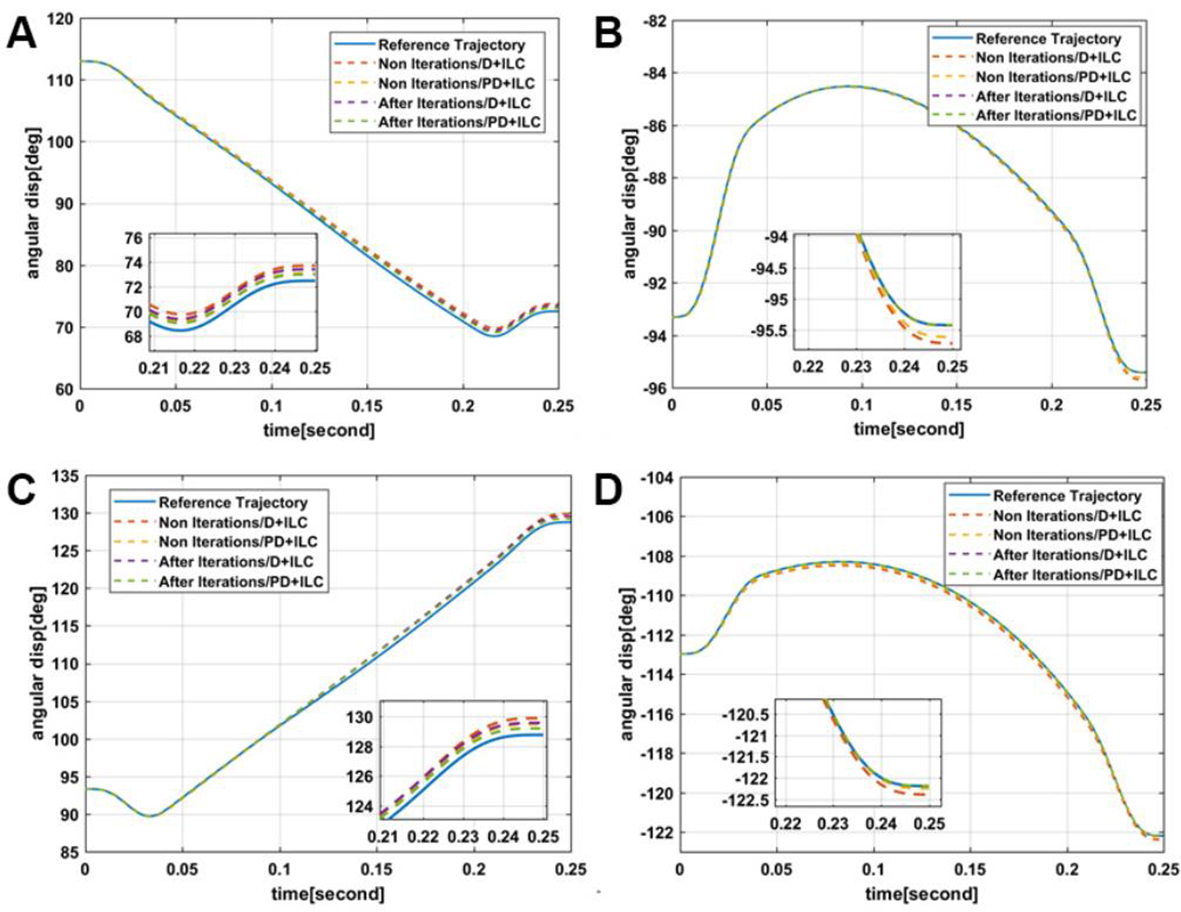Figure 5. Comparison of the actual and expected joint displacements: (A-D) Joints 1–4.

Figure 7 shows the varying tracking errors of each joint. The maximum and mean tracking errors of the two controllers are given in Table 2. As shown in Figure 7, the two controllers have similar error trends. The errors of Joints 1 and 3 increase rapidly from the beginning of the rotational motion and reach the maximum values after the complete rotation, of which the maximum values are 0.94$$^{\circ}$$ and 0.81$$^{\circ}$$ for D-ILC and 0.71$$^{\circ}$$ and 0.61$$^{\circ}$$ for PD-ILC, respectively. The other two joints can achieve good performances after iterative learning, with the maximum errors approximating to zero, as shown in Table 2.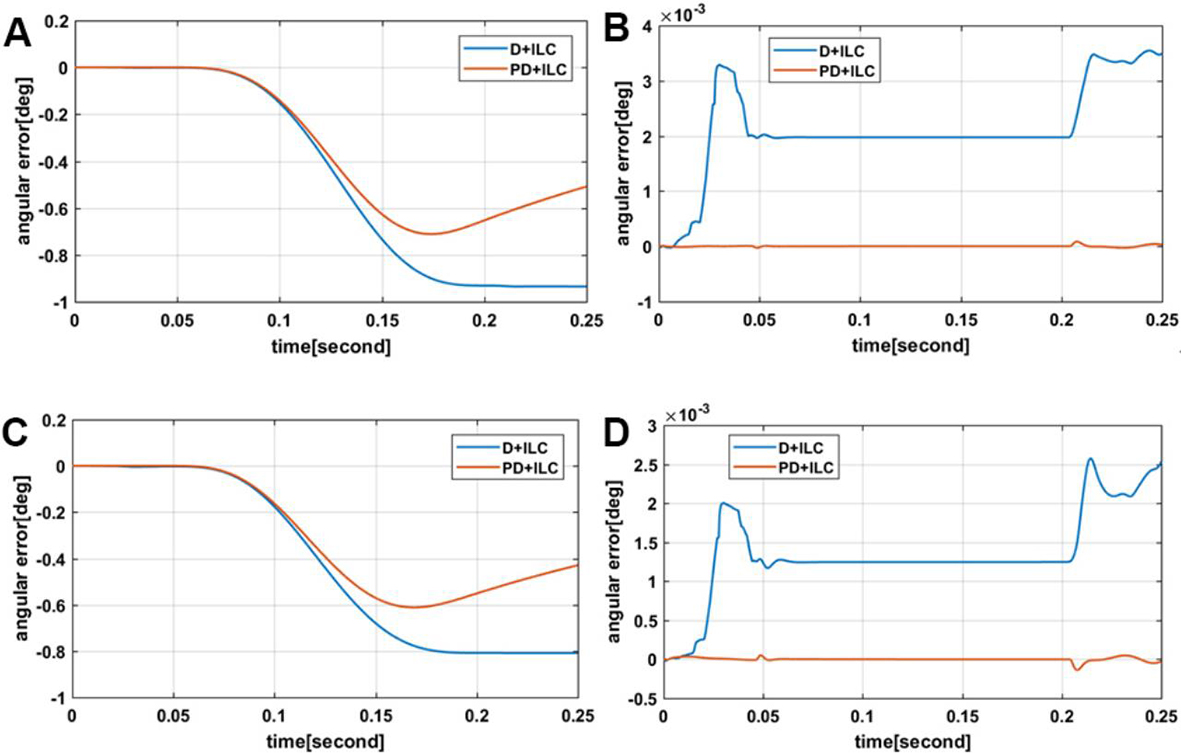Figure 7. Trajectory tracking errors of actuated joints with D-ILC and PD-ILC laws after learning iterations: (A-D) Joints 1–4.

Table 2

The tracking errors of joints under D-ILC and PD-ILC law

 Max Error (deg) Mean Error (deg) Joint $$i$$ controller 1 2 3 4 1 2 3 4 D-ILC 0.94 0.0035 0.81 0.0026 0.33 0.0018 0.30 0.0016 PD-ILC 0.71 0.0021 0.61 0.0016 0.27 0.0004 0.24 0.0003

Although the proposed control law presents superior performance compared to D-ILC, especially for Joints 2 and 4, the convergence errors of the others are still quite large. The reason lies in two aspects. On the one hand, the rotation of the robot end-effector is generated through the relative movement of the upper platform by Limbs 1 and 3, while the remaining limbs keep static. Simultaneously, the rotational motion is not continuous with the previous; therefore, the learned information cannot compensate for the errors well. On the other hand, the ILC algorithm is equivalent to an integrator along the iterative axis. It cannot guarantee that the learned information is all useful, which will lead to large errors.

Figure 8 shows the error convergence curves, where the system errors gradually converge with the increasing iterations. It can be seen that the angular displacement errors have significantly reduced after the first learning. The joint errors will become constant after the fourth iteration under the PD-ILC controller. On the contrary, there is an increase under the D-ILC law in the process of convergence.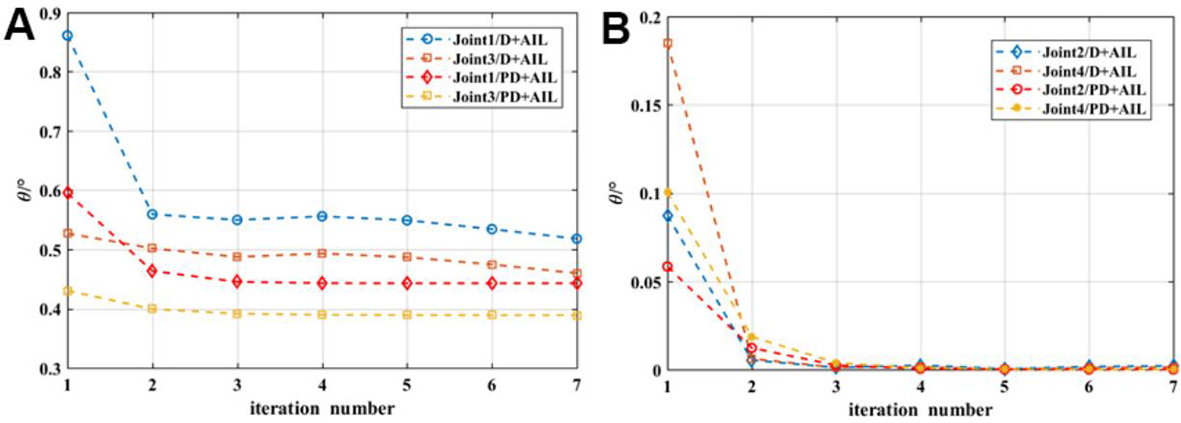Figure 8. The varying RMSEs along with the iterations: (A) Joints 1 and 3; and (B) joints 2 and 4.

The RMSEs for Joints 2 and 4 tend to zero from 0.0556$$^{\circ}$$ and 0.0952$$^{\circ}$$ under the PD-ILC law, while the errors under the D-ILC control law converge from 0.0874$$^{\circ}$$ and 0.1850$$^{\circ}$$ to 0.0021$$^{\circ}$$ and 0.0013$$^{\circ}$$ , respectively. The RMSEs for Joints 1 and 3 eventually converge to 0.3963$$^{\circ}$$ and 0.3473$$^{\circ}$$ for PD-ILC and 0.5180$$^{\circ}$$ and 0.4597$$^{\circ}$$ for D-ILC, respectively. It can be clearly seen that PD-ILC presents superior performance compared to the D-ILC controller.

### 5.2. Robustness analysis

In the real robotic application, the changes of the external environment and the existence of uncertain parameters make it difficult for the system to achieve the ideal state. For instance, the uncertain parameters of the robot and the joint friction in the movement will cause interference. In view of the external environment of such a robotic system, unpredictable and random disturbances may occur; therefore, the following two forms of disturbance are defined:

\begin{align} \begin{cases} \vec{\mathit{\boldsymbol{\tau}}}_\rm{dis} =2\sin ({\vec{{\mathit{\boldsymbol{q}}}}_{d}})-\sin ({\vec{\dot{{\mathit{\boldsymbol{q}}}}}_{d}}) \\ \vec{\mathit{\boldsymbol{\tau}}}_\rm{dis\_re} =\lambda \sin (\alpha t+\varphi) \\ \end{cases} \end{align}

where $$\vec{\bm \tau}_\rm{dis\_re}$$ represents the repetitive disturbance torque and $$\vec{\bm \tau}_\rm{dis}$$ is non-repetitive disturbance torque, $$\lambda$$ being the repetitive disturbance gain. Moreover, $$\alpha$$ and $$\varphi$$ stand for the angular frequency and phase, respectively. Figure 9 shows the corresponding repetitive and non-repetitive disturbance torques of each joint.

Figure 10 depicts the error convergences with the increasing iterations when considering the disturbance. Compared to Figure 8, the finally converged errors of the proposed ILC are larger, compared to the error convergences without disturbance, which shows that the influence of the disturbance onto the motion accuracies of the joints cannot be ignored. The maximum and mean tracking errors with disturbance and without disturbance are given in Table 3. It is noteworthy that, when the system has external disturbances, the joint errors of the robot can still converge to a certain range after iterative learning, which indicates the robustness of the proposed control law.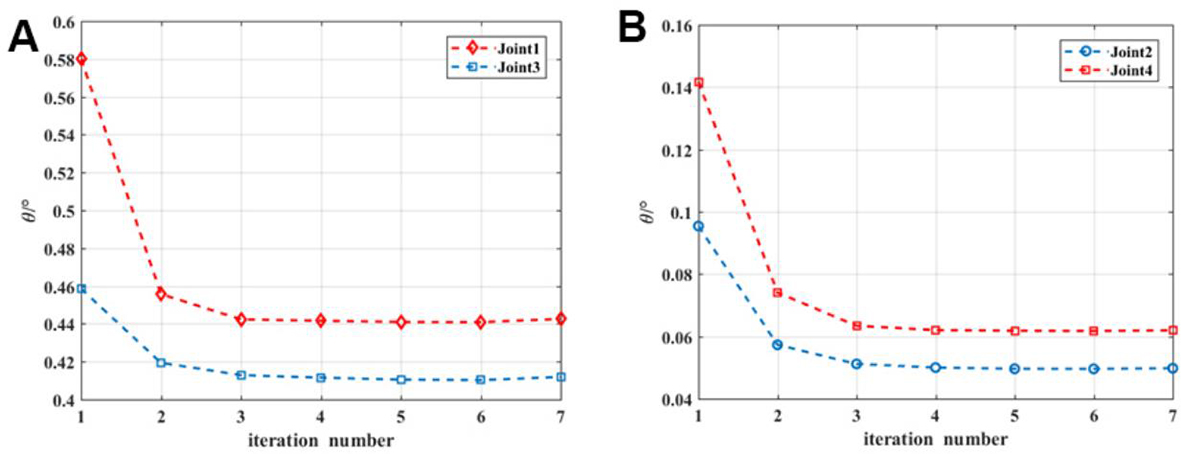Figure 10. The varying RMSE with the increasing iterations: (A) Joints 1 and 3; and (B) joints 2 and 4.

Table 3

The tracking errors under non-disturbance and disturbance

 Max Error (deg) Mean Error (deg) Joint $$i$$ 1 2 3 4 1 2 3 4 Non-disturbance 0.71 0.0021 0.61 0.0016 0.27 0.0004 0.24 0.0003 Disturbance 0.76 0.087 0.68 0.089 0.32 0.039 0.30 0.057

### 5.3. Overall performance analysis

To evaluate the overall performance of ILC in the workspace, multiple pick-and-place trajectories are selected, as displayed in Figure 11. Table 4 shows the maximum and mean tracking errors of the joints along with different paths, from which it can be seen that all the joint errors along with the selected trajectories can converge to a value after iterative learning, and the converged magnitudes are quite close.

Table 4

The tracking errors along with different paths within the workspace

 Max Error (deg) Mean Error (deg) Joint $$i$$ Path $$i$$ 1 2 3 4 1 2 3 4 Path 1 0.71 0.0021 0.61 0.0016 0.27 0.0004 0.24 0.0003 Path 2 0.62 0.0001 0.67 0.0001 0.22 0.00003 0.24 0.00004 Path 3 0.27 0.0023 0.76 0.0015 0.069 0.0006 0.19 0.0003 Path 4 0.53 0.0009 0.33 0.0017 0.16 0.0002 0.10 0.0004

Moreover, different working frequencies and trajectories are selected to evaluate the generalization ability of the controller. The results are listed in Tables 5 and 6, respectively. Figure 12 shows the varying RMSE for different trajectories.

Table 5

Results of different working frequencies with the proposed controller

 Max. Error (deg) Mean Error (deg) Joint $$i$$ Time $$i$$ 1 2 3 4 1 2 3 4 0.25 0.71 0.0021 0.61 0.0016 0.27 0.0004 0.24 0.0003 0.15 0.78 0.0039 0.68 0.0021 0.37 0.0013 0.33 0.0007 0.50 0.58 0.0004 0.50 0.00025 0.23 0.0001 0.20 0.00008
Table 6

Results by tracking different PPO trajectories

 Error Type Joint $$i$$ 4-5-6-7 th polynomial 5 th polynomial Max. Error (deg) Joint 1 0.7113 0.6854 Joint 2 0.0021 0.0032 Joint 3 0.6116 0.5875 Joint 4 0.0016 0.0020 Mean Error (deg) Joint 1 0.2697 0.2707 Joint 2 0.0004 0.0013 Joint 3 0.2404 0.2447 Joint 4 0.0003 0.0009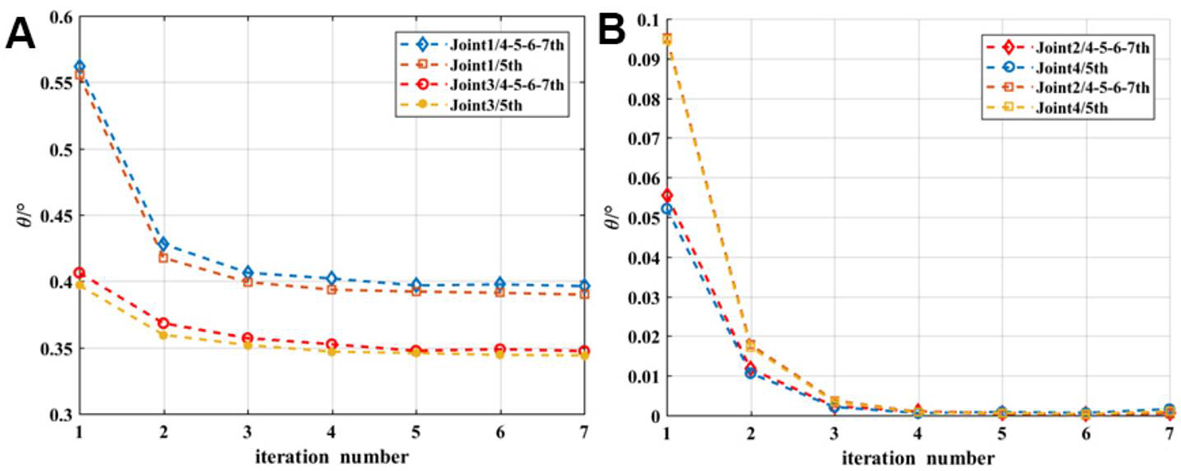Figure 12. The varying RMSEs for different trajectories: (A) Joints 1 and 3; and (B) joints 2 and 4.

From the results, it can be seen that the proposed controller shows good performance under different operating frequencies and different trajectories, meaning that the proposed control law can work effectively to track different task trajectories and have good generalization capabilities.

## 6. CONCLUSIONS

In this work, an open-closed loop PD type iterative learning control method is proposed for parallel robots to track repetitive work trajectories, thanks to its advantages of simple implementation and practicability in industrial engineering. According to the complexity and uncertainties of the working environment, two external disturbances, i.e., repetitive and non-repetitive ones, are taken into account for the model-based control design. The designed controller is compared with the D-ILC law and evaluated along with a 4-dof parallel robot, and the results show the better performance of the PD-ILC law compared with the classical D-ILC law. The test results with and without disturbances also show the robustness in terms of the trajectory tracking errors. In addition, different working frequencies and trajectories are adopted to evaluate the generalization capabilities of the controller, and the results show that the proposed PD-ILC controller has good overall performance. The developed controller can effectively work with acceptable motion errors and computation burden from the perspective of industrial engineering, which is applicable to other high-speed parallel robots of this family. In the future, the control variables will be optimized for performance improvement.

## DECLARATIONS

### Authors' contributions

Conceptualization, Methodology, Software, Writing, & editing: Li Q

Software, Data curation: Liu E

Conceptualization, Review: Cui C

Conceptualization, Methodology, Review & editing, Proofreading: Wu G

All the authors approved the submitted manuscript.

### Availability of data and materials

Not applicable.

### Financial support and sponsorship

This work was supported by Natural Science Foundation of Liaoning Province (Grant No. 20180520028).

### Conflicts of interest

The author declared that there are no conflicts of interest.

### Ethical approval and consent to participate

Not applicable.

### Consent for publication

Not applicable.

© The Author(s) 2022.

## References

• 1. Suri S, Jain A, Verma N, Prasertpoj N. SCARA Industrial Automation Robot, 2018 International Conference on Power Energy, Environment and Intelligent Control (PEEIC), 2018; p. 173-77.

• 2. Song X, Zhao Y, Jin L, Zhang P, Chen C. Dynamic feedforward control in decoupling space for a four-degree-of-freedom parallel robot. International Journal of Advanced Robotic Systems 2019;16:172988141882045.

DOI
• 3. Dang J, Ni F, Liu Y, et al. Control strategy for flexible manipulator based on feedforward compensation and fuzzy-sliding mode control. Journal of Xi'an Jiaotong University 2011;45:75-80.

• 4. Dao QT, Yamamoto SI. Modified computed torque control of a robotic Orthosis for gait rehabilitation. Annu Int Conf IEEE Eng Med Biol Soc 2018;2018:1719-22.

DOIPubMed
• 5. Su Y, Zheng C. A new nonsingular integral terminal sliding mode control for robot manipulators. International Journal of Systems Science 2020;51:1418-28.

DOI
• 6. Wu G, Zhang X, Zhu L, Lin Z, Liu J. Fuzzy sliding mode variable structure control of a high-speed parallel PnP robot. Mechanism and Machine Theory 2021;162:104349.

DOI
• 7. Uchiyama M. Formation of high-speed motion pattern of a mechanical arm by trial. T SICE 1978;14:706-12.

DOI
• 8. Arimoto S, Kawamura S, Miyazaki F. Bettering operation of Robots by learning. J Robotic Syst 1984;1:123-40.

DOI
• 9. Long Y, Du Z, Wang W. An adaptive sliding mode-like P-type iterative learning control for robot manipulators. 2014 14th International Conference on Control, Automation and Systems (ICCAS 2014).

• 10. Zhao Y, Zhou F, Wang D, et al. Path-tracking of mobile robot using feedback-aided P-type iterative learning control against initial state error. IEEE 2017; doi: 10.1109/ddcls.2017.8068138.

DOI
• 11. Bouakrif F. D-type iterative learning control without resetting condition for robot manipulators. Robotica 2011;29:975-80.

DOI
• 12. Chen Q, Lou Y. Compensated iterative learning control of industrial robots. 2018 IEEE International Conference on Real-time Computing and Robotics (RCAR); 2018. p. 52-7.

• 13. Ye Y, Tayebi A, Liu X. A unit-gain D-type iterative learning control scheme: application to a 6-dof robot manipulator. IEEE 2007; doi: 10.1109/isic.2007.4450892.

DOI
• 14. Ratcliffe JD, Hätönen JJ, Lewin PL, Rogers E, Harte TJ, Owens DH. P-type iterative learning control for systems that contain resonance. Int J Adapt Control Signal Process 2005;19:769-96.

DOI
• 15. Dong J, He B, Zhang C, Li G. Open-Closed-Loop PD Iterative Learning Control with a Variable Forgetting Factor for a Two-Wheeled Self-Balancing Mobile Robot. Complexity 2019;2019:1-11.

DOI
• 16. Sun Y, Lin H, Li ZA. Open-loop PD-Type Iterative Learning Control for a Class of Nonlinear Systems with Control Delay and Arbitrary Initial Value[J]. Measurement and Control Technology 2010;31:387-92.

• 17. Zhang L, Chen W, Liu J, Wen C. A robust adaptive iterative learning control for trajectory tracking of permanent-magnet spherical actuator. IEEE Trans Ind Electron 2016;63:291-301.

DOI
• 18. Boudjedir CE, Boukhetala D, Bouri M. Iterative learning control of a parallel delta robot. In: Chadli M, Bououden S, Ziani S, Zelinka I, editors. Advanced Control Engineering Methods in Electrical Engineering Systems. Cham: Springer International Publishing; 2019. p. 72-83.

• 19. Ma R, Zhang G. Iterative learning tracking control for a class of MIMO nonlinear time-varying systems. International Journal of Modelling Identification and Control 2017; 27(4): 271. Available from: https://www.inderscienceonline.com/doi/pdf/10.1504/IJMIC.2017.084721[Last accessed on 18 Mar 2022].

• 20. Ouyang P, Zhang W, Gupta MM. An adaptive switching learning control method for trajectory tracking of robot manipulators. Mechatronics 2006;16:51-61.

DOI
• 21. Roveda L, Forgione M, Piga D. Robot control parameters auto-tuning in trajectory tracking applications. Control Engineering Practice 2020;101:104488.

DOI
• 22. Liu S, Meng D, Cheng L, et al. An iterative learning controller for a cable-driven hand rehabilitation robot. IEEE 2017; doi: 10.1109/IECON.2017.8216989.

DOI
• 23. Yang XF, Fan XP, Yang SY, et al. Open and closed loop PD-type iterative learning control of nonlinear system and its application in robots. Journal of Changsha Railway Institute 2002:78-84.

DOIPubMed
• 24. Wu G, Cui C, Wu G. dynamic modeling and torque feedforward based optimal fuzzy PD control of a high-speed parallel manipulator. jrc 2021;2.

DOI

Li Q, Liu E, Cui C, Wu G. An open-closed-loop iterative learning control for trajectory tracking of a high-speed 4-dof parallel robot. Intell Robot 2022;2(1):89-104. http://dx.doi.org/10.20517/ir.2022.02

Views
767
168
Citations0
0

3

• Cited:0